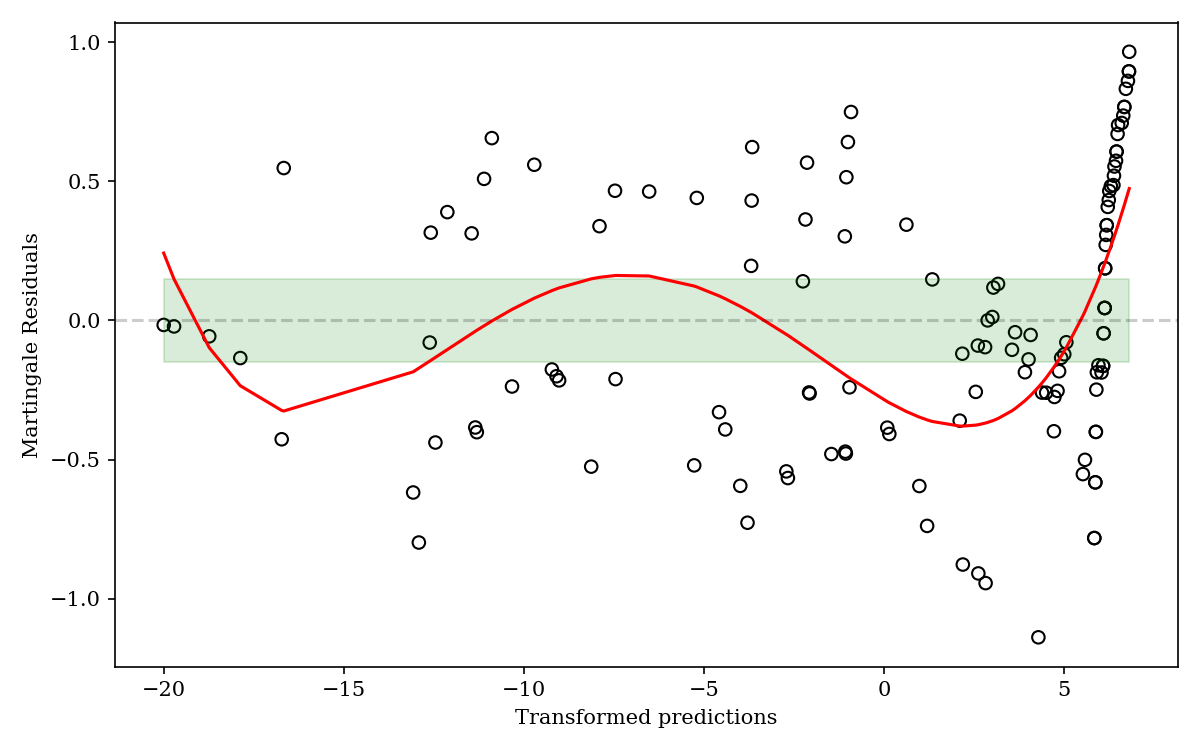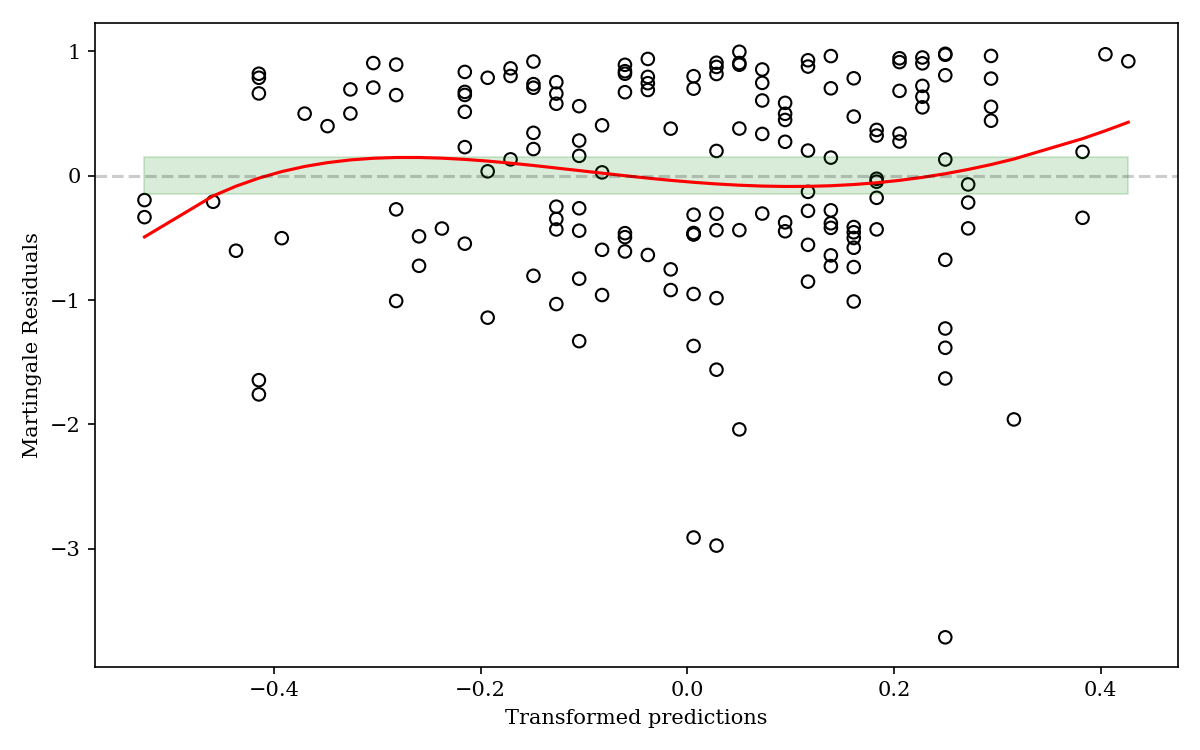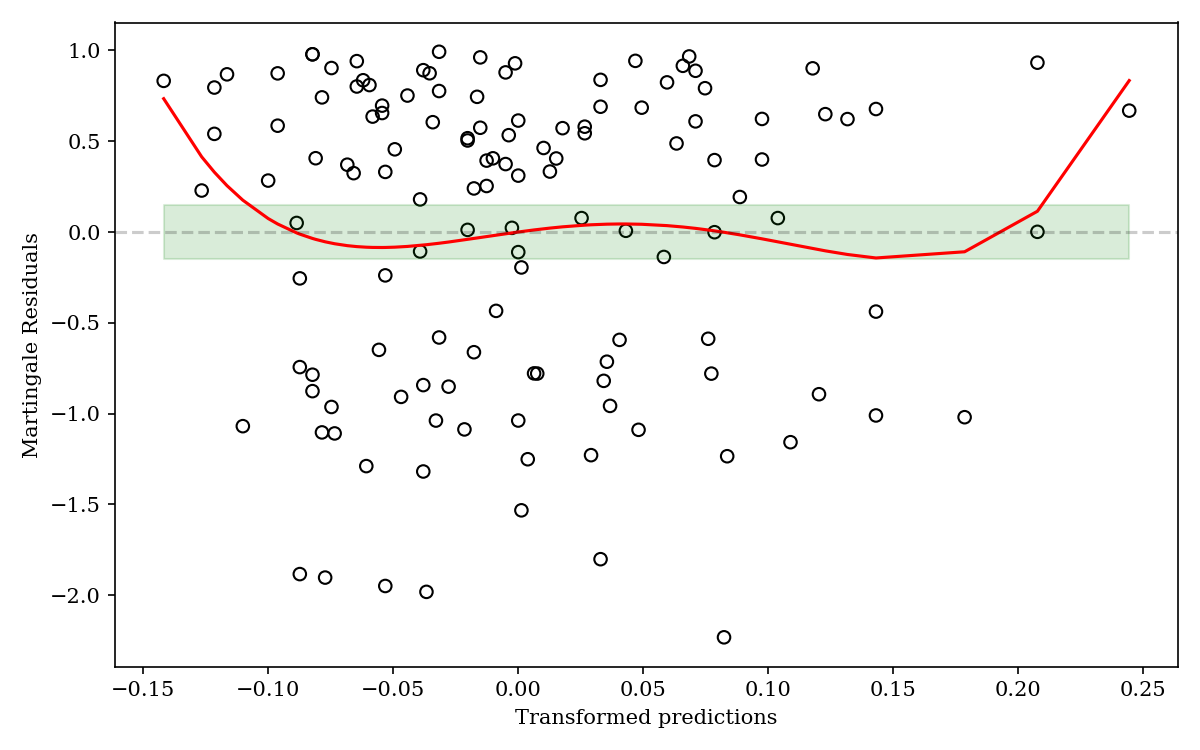# Linearity assessment in Cox regression analysis

Modified on Thu, 08 Jul 2021 at 01:58 PM

Cox model implies that there is a linear relationship between the log-hazard and the continuous covariates (explanatory variables). Thus, the assessment of this assumption is crucial for the veracity of the results and their interpretation.

To assess linearity for the Cox model we plot the martingale residuals. A residual can be viewed as the difference between the observed number of deaths for a subject and the expected numbers based on the fitted Cox model.

To check this crucial assumption in an easy graphical way, the problem is mainly reduced to answering the following question: Is the red line within the green zone ?

A resulting plot that does present a non steady, a non generally horizontal pattern with clear deviations from the green line is a sign of the linearity assumption violation.

The chart below shows an example of such violation :We clearly see a flagrant deviation from the green zone and more importantly a non linear shape of the “lowess” red line. This clearly violates the linearity assumption.

For valid linearity assumption plots, we should look at the ones below:We can argue that in those valid cases the charts still have some non-linearity, especially in the edges, but we clearly see that the “lowess” red line is generally within the green zone in the areas where we have a large number of scatter observations.

Thus, as long as the red line presents slight deviations from the horizontal dashed line and the green zone, in the areas where we have sufficient number of observations, the linearity assumption is not violated and the Cox model results interpretation is more accurate.